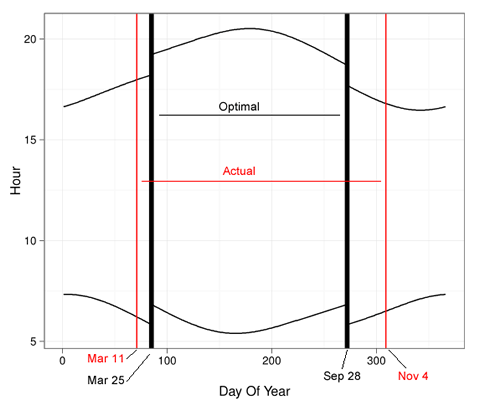# Follow-up: So … daylight savings time does not minimize variance in sunrises

December 3, 2012
By

Want to share your content on R-bloggers? click here if you have a blog, or here if you don't.

NOT SURE WHY DAYLIGHT SAVINGS TIME IS WHEN IT ISLast week we posted a nice theory about daylight savings time, in particular, that its dates were chosen to reduce variance in the time of sunrise. It looked plausible from the graph.

We were talking to our Microsoft Research colleague Jake Hofman who suggested “why don’t you just find the optimal dates to change the clock by one hour?” So we did. We got the times of sunrise for New York City from here, threw them into R, and optimized.

The result was surprising. The dates of daylight savings time do not come close to minimizing variance in sunrise. If they did, in 2012, DSL would have started on March 25th and ended on September 28th. In actuality, it started on March 11th and ended on November 4th. For NYC, daylight savings time starts too early and ends too late to minimize variance in sunrise. In the heatmap above, the higher the variance, the bluer the squares. The variance minimizing dates are shown in black, and the actual ones in red. The same color coding is used in the plot below, which also shows how the hours would shift if the variance minimizing dates were chosen (see last week’s post for how they actually change).So what then is the logic behind DSL? We’re not quite sure. There are some leads in this article. We also learned that the US lengthened DSL in 2007 as it believes it that DSL saves energy, but it is not clear that it does.

If you want to play with this, the data are here: Sunrise and Sunset data for New York City in 2012. The source of the data is here.

``` library(ggplot2) #data from http://aa.usno.navy.mil/data/docs/RS_OneYear.php setwd("C:/Dropbox/Projects/Sunrise/") df=read.table("nyc_sunrise.txt",colClasses="character") temp_times=unlist(df[,c(paste("V",seq(2,24,2),sep=""), paste("V",seq(3,25,2),sep=""))]) l=length(temp_times)/2 df=data.frame(day=1:31,stime=temp_times, sun=c(rep("rise",l),rep("set",l))) rm(temp_times,l) hour=as.numeric(substr(df\$stime,1,2)) minute=as.numeric(substr(df\$stime,3,4))/60 df\$time=hour+minute df=subset(df,!is.na(df\$time)) df\$day_of_year=1:(nrow(df)/2) p=ggplot(data=subset(df,sun=="rise"),aes(x=day_of_year,y=time)) p=p+geom_line() p=p+geom_line(data=subset(df,sun=="set"),aes(x=day_of_year,y=time)) p zerovec=function(i,j){ c(rep(0,i-1), rep(1,j-i+1), rep(0,len-j))} currvec=df[df\$sun=="rise","time"] len=length(currvec) get_var=function(i,j) { var(currvec + zerovec(i,j))} vget_var=Vectorize(get_var) result=expand.grid(spring_forward=45:125,fall_back=232:312) result=subset(result,spring_forward result\$var=with(result,vget_var(spring_forward,fall_back)) resout=result[which.min(result\$var),] resout #Heatmap p =ggplot(data=result, aes(spring_forward, fall_back)) + geom_tile(aes(fill = var), colour = "white") + scale_fill_gradient(low = "white", high = "steelblue") p=p+geom_vline(xintercept=as.numeric(resout))+ geom_hline(yintercept=as.numeric(resout)) p=p+geom_vline(xintercept=71,color="red")+ geom_hline(yintercept=309,color="red") p=p+ylab("Fall Back Day of Year\n")+theme_bw() p=p+xlab("\nLeap Forward Day of Year")+opts(legend.position="none") p ggsave("heatmap.pdf",p,width=6) #For 2012, optimal spring forward day (85)is 03/25/2012 #For 2012, optimal fall back day (272) is 09/28/2012 #Actual DSL start was 3/11/2012 (71) #Actual DSL end was 11/4/2012 (309) p=ggplot(data=subset(df,sun=="rise"), aes(x=day_of_year,y=time+zerovec(85,272))) p=p+geom_line() p=p+geom_line(data=subset(df,sun=="set"), aes(x=day_of_year,y=time+zerovec(85,272))) p=p+geom_vline(xintercept=85,lwd=2)+ geom_vline(xintercept=272,lwd=2) p=p+geom_vline(xintercept=71,color="red")+ geom_vline(xintercept=309,color="red") p=p+ylab("Hour")+theme_bw() p=p+xlab("\nDay Of Year")+opts(legend.position="none") p ggsave("timeshift.pdf",p,width=6) ```

Figures created with Hadley Wickham’s ggplot2The post Follow-up: So … daylight savings time does not minimize variance in sunrises appeared first on Decision Science News.

R-bloggers.com offers daily e-mail updates about R news and tutorials about learning R and many other topics. Click here if you're looking to post or find an R/data-science job.
Want to share your content on R-bloggers? click here if you have a blog, or here if you don't.